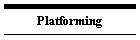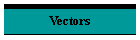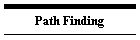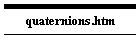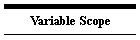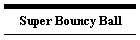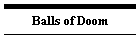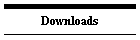# Coordinates in two dimensions

The position of an object may typically be defined by its x and y coordinates, where x represents a horizontal distance from the leftmost edge of the game area and y represents a vertical distance from the top edge of the game area.

You should note that in mathematics Y coordinates are usually measured upwards, whereas in Gamemaker Y coordinates are measured downwards with zero at the top of the play area.

# Vector

A vector is typically a set of coordinates defining a move from one place to another. A position can be considered a vector, it's just a move from the origin (0,0) to where the object is now.

If I have two objects Object1 and Object2 then I can calculate a vector from Object1 to Object2 as

vx=object2.x-object1.x
vy=object2.y-object1.y

# Rectangular and Polar coordinates

A vector can be an x and y coordinate, but it can also be a distance and a direction.

The above vector (vx,vy) can be converted into a polar (length and direction) form using two formulae:

distance=sqrt(sqr(vx)+sqr(vy))

The formula arctan(-vy/vx) is only correct for a limited range of angles, arctan2 is valid for all angles

vy is negated due to the way Gamemaker handles angles.

Gamemaker also offers a shorter version using the point_direction and point_distance functions. If we make the start point (0,0) we get:

direction=point_direction(0,0,vx,vy)

distance=point_distance(0,0,vx,vy)

Polar form can be converted back to x and y:

or

x=lengthdir_x(distance,direction)

y=lengthdir_y(distance,direction)

# Dot Products

The dot product is a very useful operation for comparing angles without actually calculating the angle.. It has two definitions, one that is easy to calculate and another that is more understandable.

Given two vectors (ax,ay) and (bx,by) the dot product is ax*bx+ay*by

In terms of geometry the dot product is obtained by multiplying the length of one vector by the length of the other vector and by the cosine of the angle between them. If both vectors are unit length (length=1) then the dot product is simply the cosine of the angle between them.

Gamemaker Studio offers us dedicated functions for calculating dot products:dot_product(x1, y1, x2, y2)dot_product_normalised(x1, y1, x2, y2)Dot product normalised will first scale the two vectors to unit length before calculating the product so the result lies between 1 and -1.

Given the above the dot product offers us a way of measuring whether two vectors point in the same direction without having to convert them to angles. dot_product_normalised is particularly good as it eliminates the vectors' length.

# Cross Products

Strictly speaking the cross product is not valid for two dimensions but if we imagine a third z dimension pointing up out of the screen then they can still be put to some use in 2D.

Given two vectors the cross product in three dimensions is a vector at right angles to the two vectors and with a length equal to the product of the lengths of the two vectors multiplied by the sine of the angle between them.

The cross product of (ax,ay,az) and (bx,by,bz) is the vector (ay*bz-az*by,az*bx-ax*bz,ax*by-ay*bx)

If we start with 2D vectors then az and bz must be zero so the x and y parts of the result must be zero. The result is only z=(ax*by-ay*bx).

This could be calculated by misusing the dot product function with the parameters rearranged to (x1,y1, -y2,x2)

Since we are using Gamemaker coordinates it follows that if the second vector is clockwise of the first then z is positive but if the second vector is anticlockwise then z is negative.